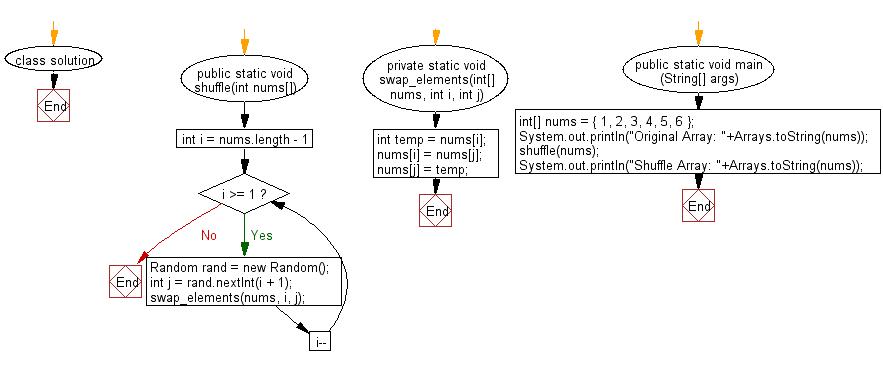﻿ Java exercises: Shuffle a given array of integers - w3resource# Java Array Exercises: Shuffle a given array of integers

## Java Array: Exercise-60 with Solution

Write a Java program to shuffle a given array of integers.

Example:
Input :
nums = { 1, 2, 3, 4, 5, 6 }
Output:
Shuffle Array: [4, 2, 6, 5, 1, 3]

Sample Solution:

Java Code:

``````import java.util.Arrays;
import java.util.Random;

class solution
{
public static void shuffle(int nums[])
{
for (int i = nums.length - 1; i >= 1; i--)
{
Random rand = new Random();

int j = rand.nextInt(i + 1);

swap_elements(nums, i, j);
}
}
private static void swap_elements(int[] nums, int i, int j) {
int temp = nums[i];
nums[i] = nums[j];
nums[j] = temp;
}
public static void main (String[] args)
{
int[] nums = { 1, 2, 3, 4, 5, 6 };
System.out.println("Original Array: "+Arrays.toString(nums));
shuffle(nums);
System.out.println("Shuffle Array: "+Arrays.toString(nums));
}
}
```
```

Sample Output:

```Original Array: [1, 2, 3, 4, 5, 6]
Shuffle Array: [4, 2, 6, 5, 1, 3]
```

Flowchart:Java Code Editor:

Improve this sample solution and post your code through Disqus

What is the difficulty level of this exercise?

Test your Programming skills with w3resource's quiz.

﻿

## Java: Tips of the Day

countOccurrences

Counts the occurrences of a value in an array.

Use Arrays.stream().filter().count() to count total number of values that equals the specified value.

```public static long countOccurrences(int[] numbers, int value) {
return Arrays.stream(numbers)
.filter(number -> number == value)
.count();
}
```

Ref: https://bit.ly/3kCAgLb